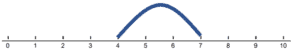• ###### Complete the Math Sentence

These Number Line Addition worksheets are designed to help students develop an appreciation for the number line as a visual tool to aid in addition problems. The worksheets include a variety of creative and engaging exercises to help students build their math skills and confidence in using the number line.

Each worksheet in the series includes a mix of problems with varying degrees of difficulty, designed to challenge and engage students at different skill levels. The worksheets ask students to solve simple addition problems with single-digit numbers using the number line. They also ask students to figure out the addends and sum in an addition equation by observing how its number line was used through drawing hops across it.

This Number Line Addition worksheet series is suitable for students in grades 1 to 3. It can be used in the classroom as a supplement to the math curriculum or at home as a way for parents to help their children practice and reinforce their math skills.

Overall, these Number Line Addition worksheets provide a comprehensive and engaging way for students to develop their math skills and confidence in using the number line as a visual tool to aid in addition problems. With these worksheets, students can build a solid foundation in math and develop the skills they need to succeed in higher-level math courses.

Number lines are a helpful visual tool that can assist in learning addition, especially for students who are just beginning to grasp the concept. Number lines provide a linear representation of numbers, which can help students understand the relationship between numbers and develop a better intuition for addition. Here are some ways number lines can help you learn to add:

Understanding Number Sequence – Number lines help students recognize the sequence and order of numbers, which is essential when learning addition.

Visualizing Addition – When adding two numbers, a number line can be used to show the process of moving along the line by the value of the second number. This visualization can help students understand how addition combines numbers to form a larger value.

Developing Number Sense – Using a number line can help students develop a sense of relative size and distance between numbers, which can make addition problems easier to conceptualize and solve.

Introducing Skip Counting – Number lines can help students learn skip counting (counting by 2s, 5s, or 10s, for example), which is a valuable skill for addition and multiplication.

Here’s how to use a number line to add two numbers:

Example – Add 4 + 3 using a number line.

Draw a Number Line – Draw a horizontal line and mark the numbers from 0 to at least the sum of the two numbers you want to add (in this case, 0 to 7 or higher).

Locate the First Number – Place a marker or your finger on the first number you want to add (4 in this example) on the number line.

Count Forward – Move your marker or finger along the number line by the value of the second number you’re adding (3 in this example). Count the steps as you go (1, 2, 3).Identify the Sum – When you’ve moved the correct number of steps, check the number where your marker or finger has landed. This is the sum of the two numbers you added (7 in this example).

So, 4 + 3 = 7, as shown on the number line.

By using number lines to practice addition, students can develop a better understanding of the concept and build a strong foundation for more advanced mathematical concepts.